# Container with water

The weight of a container with water is 2.48 kg. If the cast 75% water, the container with water has a weight of 0.98 kg. Determine the weight of the empty container. How much water was originally in the container?

m =  0.48 kg
v =  2 kg

### Step-by-step explanation:

m + v = 2.48
m+(1-75/100)•v = 0.98

m+v = 2.48
100m+25v = 98

m = 12/25 = 0.48
v = 2

Our linear equations calculator calculates it.Did you find an error or inaccuracy? Feel free to write us. Thank you!Tips to related online calculators
Do you have a system of equations and looking for calculator system of linear equations?
Tip: Our volume units converter will help you with the conversion of volume units.

## Related math problems and questions:

• Water containerContainer with water weighs 1.48 kg. When we cast 75% of water container of water weight 0.73 kg. How heavy is an empty container?
• Barrel 3Barrel with water weights 118 kg. When we get off 75% of water, it weights 35 kg. How many kg has an empty barrel?
• Barrel with liquid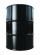Barrel with grain weight 297 kg. When it shed 48% of grain, ha weight 174 kg. What is the weight of empty barrel?
• Barrel 4Barrel of water weighs 63 kg. After off 75% water, the weight of the barrel with water is 21 kg. How many kg weigh empty barrel and how many kgs water in it?
• WeightlifterWeightlifter lifted 75% of its weight. Determine how much weight lifted when he weighs 132 kg.
• Barrel 2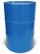Empty barrel weighs 7 kg. When it filled with water up to 45% height, weighs 60 kg. How heavy is barrel full of water?
• The water barrel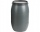The water barrel weighs 122 kg. If we pour 75% of the water out of it, it will weigh 35 kg. What is the weight of the barrel?
• Water in vegetables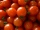Tomatoes in the store contain 99% water. After being transported to the shop, they were slightly dried and contained only 98% of water. How many kgs of tomatoes are in the store if there were 300 kg in stock?`
• Barrel with water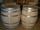The water barrel has a weight of 52 kg. When we pour a quarter of the water, the weight of the barrel is reduced to 40 kg. Determine the weight of the empty barrel.
• Camel and water84% of the camel's weight is water. After drinking, its weight increased to 832 kg, and water accounted for 85% of its weight. How much did it weigh before drinking?
• Barrel of oilBarrel of oil weighs 283 kg. When it mold 26% oil, weighed 216 kg. What is the mass of the empty barrel?
• Mushrooms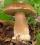Fresh mushrooms contain 94% water, dried 14%. How many kg of fresh mushrooms is needed to collect to get 10 kg dried?
• SpiritFrom 55% and 80% spirit we would like to produce 0.2 kg of 60% spirit. How many of them we must use in a solution?
• Water wessel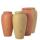The vessel containing water has a weight 19 kg. After pouring half the water its weigth 11 kg. What is the weight of the empty vessel?
• Trio weight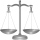Adelka, Barunka, and Cecilka are weight in pairs. Adelka with Barunka weighs 98 kg, Barunka with Cecilka 90 kg and Adelka with Cecilka 92 kg. How much does each of them weigh?
• Cargo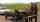The weight of the cargo car is 761 kg. The weight of the car is 23% of the total weight of car+cargo. What is the weight of the cargo load?
• Empty canisterThe canister is filled with oil weight 17 kg. If filled only half weight 9 kg. What weight does my empty canister have?